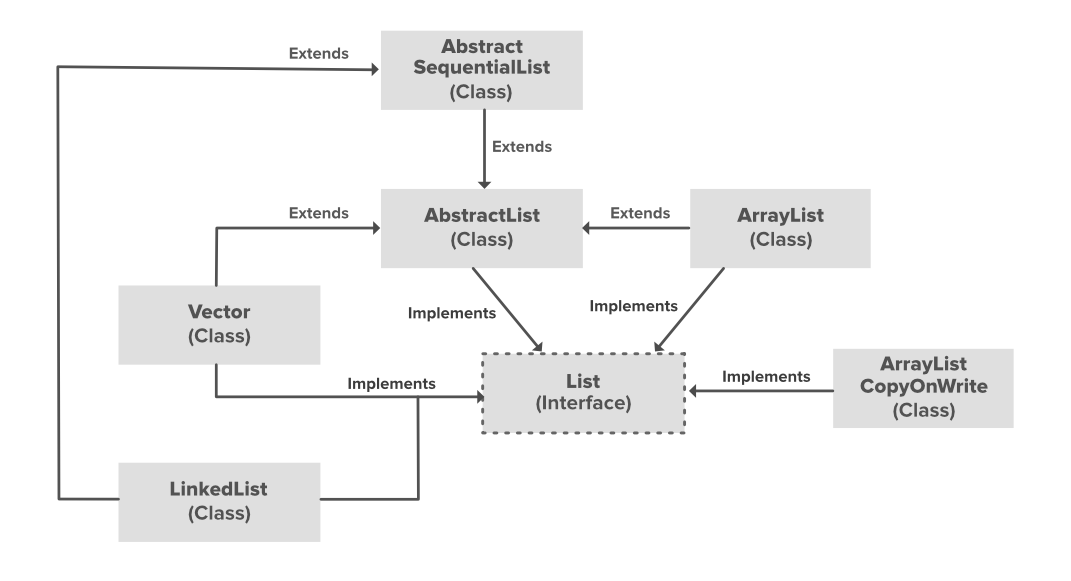• Difficulty Level : Easy
• Last Updated : 13 May, 2022

Linked List is a part of the Collection framework present in java.util package. This class is an implementation of the LinkedList data structure which is a linear data structure where the elements are not stored in contiguous locations and every element is a separate object with a data part and address part. The elements are linked using pointers and addresses. Each element is known as a node. Due to the dynamicity and ease of insertions and deletions, they are preferred over the arrays. It also has a few disadvantages like the nodes cannot be accessed directly instead we need to start from the head and follow through the link to reach a node we wish to access.Since a LinkedList acts as a dynamic array and we do not have to specify the size while creating it, the size of the list automatically increases when we dynamically add and remove items. And also, the elements are not stored in a continuous fashion. Therefore, there is no need to increase the size. Internally, the LinkedList is implemented using the doubly linked list data structure. The main difference between a normal linked list and a doubly LinkedList is that a doubly linked list contains an extra pointer, typically called the previous pointer, together with the next pointer and data which are there in the singly linked list.

In order to create a LinkedList, we need to create an object of the LinkedList class. The LinkedList class consists of various constructors that allow the possible creation of the list. The following are the constructors available in this class:

1. LinkedList(): This constructor is used to create an empty linked list. If we wish to create an empty LinkedList with the name ll, then, it can be created as:

`LinkedList ll = new LinkedList();  `

2. LinkedList(Collection C): This constructor is used to create an ordered list that contains all the elements of a specified collection, as returned by the collection’s iterator. If we wish to create a LinkedList with the name ll, then, it can be created as:

`LinkedList ll = new LinkedList(C);`

Example:

## Java

 `// Java Program to Demonstrate``// Implementation of LinkedList``// class`` ` `// Importing required classes``import` `java.util.*;`` ` `// Main class``public` `class` `GFG {`` ` `    ``// Main driver method``    ``public` `static` `void` `main(String args[])``    ``{``        ``// Creating object of the``        ``// class linked list``        ``LinkedList ll = ``new` `LinkedList();`` ` `        ``// Adding elements to the linked list``        ``ll.add(``"A"``);``        ``ll.add(``"B"``);``        ``ll.addLast(``"C"``);``        ``ll.addFirst(``"D"``);``        ``ll.add(``2``, ``"E"``);`` ` `        ``System.out.println(ll);`` ` `        ``ll.remove(``"B"``);``        ``ll.remove(``3``);``        ``ll.removeFirst();``        ``ll.removeLast();`` ` `        ``System.out.println(ll);``    ``}``}`
Output:
```[D, A, E, B, C]
[A]```In the above illustration, AbstractList, CopyOnWriteArrayList, and the AbstractSequentialList are the classes that implement the list interface. A separate functionality is implemented in each of the mentioned classes. They are:

1. AbstractList: This class is used to implement an unmodifiable list, for which one needs to only extend this AbstractList Class and implement only the get() and the size() methods.
2. CopyOnWriteArrayList: This class implements the list interface. It is an enhanced version of ArrayList in which all the modifications(add, set, remove, etc.) are implemented by making a fresh copy of the list.

## Performing Various Operations on LinkedList

2. Updating elements
3. Removing elements
4. Iterating over elements

Let us see how to perform some basic operations on LinkedList to understand it better as follows:

In order to add an element to an ArrayList, we can use the add() method. This method is overloaded to perform multiple operations based on different parameters. They are:

• add(int index, Object): This method is used to add an element at a specific index in the LinkedList.

Example:

## Java

 `// Java program to add elements ``// to a LinkedList``   ` `import` `java.util.*; ``   ` `public` `class` `GFG { ``   ` `    ``public` `static` `void` `main(String args[]) ``    ``{ ``        ``LinkedList ll = ``new` `LinkedList<>(); ``   ` `        ``ll.add(``"Geeks"``); ``        ``ll.add(``"Geeks"``); ``        ``ll.add(``1``, ``"For"``); ``   ` `        ``System.out.println(ll); ``    ``} ``}`
Output:
`[Geeks, For, Geeks]`

Operation 2: Changing Elements

After adding the elements, if we wish to change the element, it can be done using the set() method. Since a LinkedList is indexed, the element which we wish to change is referenced by the index of the element. Therefore, this method takes an index and the updated element which needs to be inserted at that index.

Example:

## Java

 `// Java program to change elements ``// in a LinkedList ``   ` `import` `java.util.*; ``   ` `public` `class` `GFG { ``   ` `    ``public` `static` `void` `main(String args[]) ``    ``{ ``        ``LinkedList ll = ``new` `LinkedList<>(); ``   ` `        ``ll.add(``"Geeks"``); ``        ``ll.add(``"Geeks"``); ``        ``ll.add(``1``, ``"Geeks"``); ``   ` `        ``System.out.println(``"Initial LinkedList "` `+ ll); ``   ` `        ``ll.set(``1``, ``"For"``); ``   ` `        ``System.out.println(``"Updated LinkedList "` `+ ll); ``    ``} ``} `
Output:
```Initial LinkedList [Geeks, Geeks, Geeks]

Operation 3: Removing Elements

In order to remove an element from a LinkedList, we can use the remove() method. This method is overloaded to perform multiple operations based on different parameters. They are:

• remove(Object): This method is used to simply remove an object from the LinkedList. If there are multiple such objects, then the first occurrence of the object is removed.
• remove(int index): Since a LinkedList is indexed, this method takes an integer value which simply removes the element present at that specific index in the LinkedList. After removing the element and the indices of elements are updated so do the object of LinkedList is updated giving a new List after the deletion of element/s.

Example:

## Java

 `// Java program to remove elements ``// in a LinkedList``   ` `import` `java.util.*; ``   ` `public` `class` `GFG { ``   ` `    ``public` `static` `void` `main(String args[]) ``    ``{ ``        ``LinkedList ll = ``new` `LinkedList<>(); ``   ` `        ``ll.add(``"Geeks"``); ``        ``ll.add(``"Geeks"``); ``        ``ll.add(``1``, ``"For"``); ``   ` `        ``System.out.println( ``            ``"Initial LinkedList "` `+ ll); ``   ` `        ``ll.remove(``1``); ``   ` `        ``System.out.println( ``            ``"After the Index Removal "` `+ ll); ``   ` `        ``ll.remove(``"Geeks"``); ``   ` `        ``System.out.println( ``            ``"After the Object Removal "` `+ ll); ``    ``} ``} `
Output:
```Initial LinkedList [Geeks, For, Geeks]
After the Index Removal [Geeks, Geeks]
After the Object Removal [Geeks]```

There are multiple ways to iterate through LinkedList. The most famous ways are by using the basic for loop in combination with a get() method to get the element at a specific index and the advanced for-loop.

Example:

## Java

 `// Java program to iterate the elements ``// in an LinkedList``   ` `import` `java.util.*; ``   ` `public` `class` `GFG { ``   ` `    ``public` `static` `void` `main(String args[]) ``    ``{ ``        ``LinkedList ll ``            ``= ``new` `LinkedList<>(); ``   ` `        ``ll.add(``"Geeks"``); ``        ``ll.add(``"Geeks"``); ``        ``ll.add(``1``, ``"For"``); ``   ` `        ``// Using the Get method and the ``        ``// for loop ``        ``for` `(``int` `i = ``0``; i < ll.size(); i++) { ``   ` `            ``System.out.print(ll.get(i) + ``" "``); ``        ``} ``   ` `        ``System.out.println(); ``   ` `        ``// Using the for each loop ``        ``for` `(String str : ll) ``            ``System.out.print(str + ``" "``); ``    ``} ``} `
Output:
```Geeks For Geeks
Geeks For Geeks```

My Personal Notes arrow_drop_up